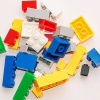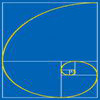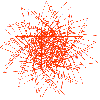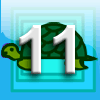# Resources tagged with: Fibonacci sequence

Filter by: Content type:
Age range:
Challenge level:

### There are 18 results

Broad Topics > Patterns, Sequences and Structure > Fibonacci sequence### Fibonacci Surprises

##### Age 11 to 14 Challenge Level:

Play around with the Fibonacci sequence and discover some surprising results!### Gnomon Dimensions

##### Age 14 to 16 Challenge Level:

These gnomons appear to have more than a passing connection with the Fibonacci sequence. This problem ask you to investigate some of these connections.### Paving Paths

##### Age 11 to 14 Challenge Level:

How many different ways can I lay 10 paving slabs, each 2 foot by 1 foot, to make a path 2 foot wide and 10 foot long from my back door into my garden, without cutting any of the paving slabs?### Building Gnomons

##### Age 14 to 16 Challenge Level:

Build gnomons that are related to the Fibonacci sequence and try to explain why this is possible.### Fibs

##### Age 11 to 14 Challenge Level:

The well known Fibonacci sequence is 1 ,1, 2, 3, 5, 8, 13, 21.... How many Fibonacci sequences can you find containing the number 196 as one of the terms?### 1 Step 2 Step

##### Age 11 to 14 Challenge Level:

Liam's house has a staircase with 12 steps. He can go down the steps one at a time or two at time. In how many different ways can Liam go down the 12 steps?### Continued Fractions I

##### Age 14 to 18

An article introducing continued fractions with some simple puzzles for the reader.### Colour Building

##### Age 11 to 14 Challenge Level:

Using only the red and white rods, how many different ways are there to make up the other rods?### The Golden Ratio, Fibonacci Numbers and Continued Fractions.

##### Age 14 to 16

An iterative method for finding the value of the Golden Ratio with explanations of how this involves the ratios of Fibonacci numbers and continued fractions.### Got a Strategy for Last Biscuit?

##### Age 11 to 16 Challenge Level:

Can you beat the computer in the challenging strategy game?### Ordered Sums

##### Age 14 to 16 Challenge Level:

Let a(n) be the number of ways of expressing the integer n as an ordered sum of 1's and 2's. Let b(n) be the number of ways of expressing n as an ordered sum of integers greater than 1. (i) Calculate. . . .### Leonardo of Pisa and the Golden Rectangle

##### Age 7 to 16

Leonardo who?! Well, Leonardo is better known as Fibonacci and this article will tell you some of fascinating things about his famous sequence.### Stringing it Out

##### Age 14 to 16 Challenge Level:

Explore the transformations and comment on what you find.### Whirling Fibonacci Squares

##### Age 11 to 16

Draw whirling squares and see how Fibonacci sequences and golden rectangles are connected.### Fibonacci's Three Wishes 2

##### Age 7 to 14

Second of two articles about Fibonacci, written for students.##### Age 11 to 16 Challenge Level:

Using logo to investigate spirals### First Forward Into Logo 11: Sequences

##### Age 11 to 18 Challenge Level:

This part introduces the use of Logo for number work. Learn how to use Logo to generate sequences of numbers.### LOGO Challenge - Circles as Bugs

##### Age 11 to 16 Challenge Level:

Here are some circle bugs to try to replicate with some elegant programming, plus some sequences generated elegantly in LOGO.